Electric Field And Potential MCQ Level - 1

# Electric Field And Potential MCQ Level - 1 - Physics

Test Description

## 10 Questions MCQ Test Topic wise Tests for IIT JAM Physics - Electric Field And Potential MCQ Level - 1

Electric Field And Potential MCQ Level - 1 for Physics 2023 is part of Topic wise Tests for IIT JAM Physics preparation. The Electric Field And Potential MCQ Level - 1 questions and answers have been prepared according to the Physics exam syllabus.The Electric Field And Potential MCQ Level - 1 MCQs are made for Physics 2023 Exam. Find important definitions, questions, notes, meanings, examples, exercises, MCQs and online tests for Electric Field And Potential MCQ Level - 1 below.
Solutions of Electric Field And Potential MCQ Level - 1 questions in English are available as part of our Topic wise Tests for IIT JAM Physics for Physics & Electric Field And Potential MCQ Level - 1 solutions in Hindi for Topic wise Tests for IIT JAM Physics course. Download more important topics, notes, lectures and mock test series for Physics Exam by signing up for free. Attempt Electric Field And Potential MCQ Level - 1 | 10 questions in 30 minutes | Mock test for Physics preparation | Free important questions MCQ to study Topic wise Tests for IIT JAM Physics for Physics Exam | Download free PDF with solutions
 1 Crore+ students have signed up on EduRev. Have you?
Electric Field And Potential MCQ Level - 1 - Question 1

### Let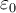denote the permittivity of vacuum, the dimensional formula of the ε0 is  let M = Mass, L = length, T = time and I = electric current. Then

Detailed Solution for Electric Field And Potential MCQ Level - 1 - Question 1

Using Coulomb law, F = 1/4πϵ0(q1q2/R2)

⇒ϵ0 = q1q2/4πFR2

Substituting the units in the equation:

So, ϵ0 = C2/N.m2

=[AT]2/MLT-2.L2

[∈0]=[M-1L-3T4A2]

Electric Field And Potential MCQ Level - 1 - Question 2

### A charge q1 exert some force on a second charge q2. If a third charge q3 is brought near q1 the force exerted by q1 on q2

Detailed Solution for Electric Field And Potential MCQ Level - 1 - Question 2

The correct answer is: Remain the same

Electric Field And Potential MCQ Level - 1 - Question 3

### An electric dipole is place in a uniform electric field. The net electric force on the dipole

Detailed Solution for Electric Field And Potential MCQ Level - 1 - Question 3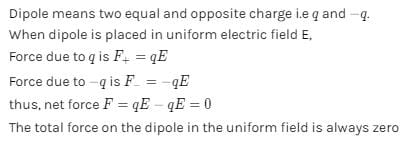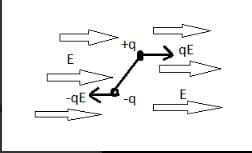Electric Field And Potential MCQ Level - 1 - Question 4

Which of the following is not true?

Detailed Solution for Electric Field And Potential MCQ Level - 1 - Question 4

The correct answer is: The electric dipole potential varies as 1/r at large distance

Electric Field And Potential MCQ Level - 1 - Question 5

A charge particle is free to move in an electric field. It will travel

Detailed Solution for Electric Field And Potential MCQ Level - 1 - Question 5

The correct answer is: Along a line of force, if its initial velocity is zero

Electric Field And Potential MCQ Level - 1 - Question 6

A uniform electric field of 100 V/m is directed at 30° with the positive x-axis as shown in figure. Find the potential difference VBA. If  OA = 2m and OB = 4m. O is the origin.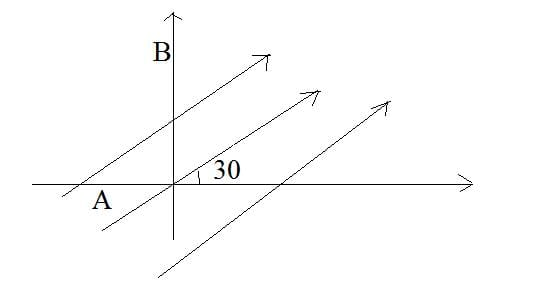Detailed Solution for Electric Field And Potential MCQ Level - 1 - Question 6

Electric field in vector form can be written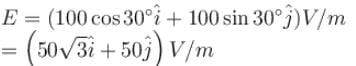A = (–2m, 0, 0)
B = (0.4m, 0)
VAB = VB – VA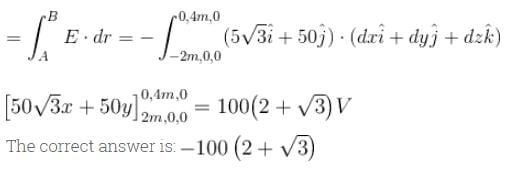Electric Field And Potential MCQ Level - 1 - Question 7

The curve represents the distribution of potential along the straight line joining. The two charge Q1 and Q2 (separated by a distance r) then which of the following statement is correct?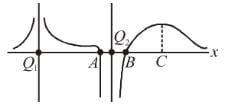(1)  |Q1| > |Q2|
(2) Q1 is positive in nature
(3)  A and B are equilibrium

Detailed Solution for Electric Field And Potential MCQ Level - 1 - Question 7

The correct answer is: 1, 2 and 3

Electric Field And Potential MCQ Level - 1 - Question 8

The number of electrons to be put on a spherical conductor of radius 0.1m to produce on electric field of 0.036NC-1 just above its surface is

Detailed Solution for Electric Field And Potential MCQ Level - 1 - Question 8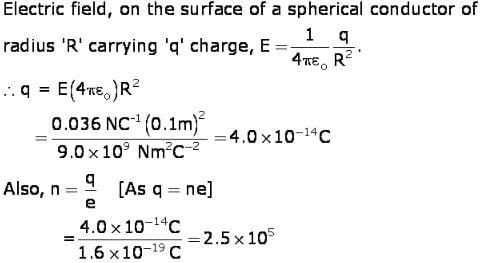Electric Field And Potential MCQ Level - 1 - Question 9

Proton is nearly 1836 times more massive than the electron. The coulomb force of repulsion between two proton separated by a finite distance is F. The electrostatic force between two electron separated by the same distance is

Detailed Solution for Electric Field And Potential MCQ Level - 1 - Question 9

Coulomb force is determined by charges not by masses.

Electric Field And Potential MCQ Level - 1 - Question 10

Two unlike charges of magnitude q are separates by a distance 2d. The potential at C point midway between them is

Detailed Solution for Electric Field And Potential MCQ Level - 1 - Question 10(Scan QR code)